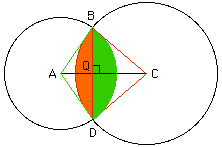```Date: Fri, 1 Nov 1996 15:14:12 -0600 (CST)

Name:	Lynda
Level:	Secondary
```

Question:
How do I find the area common to two intersecting circles of radii 8 ft and 10 ft if their common chord is 10 ft long?

Hi again Lynda

If you look at the larger circle in the diagram you can find the area I have shaded orange as the difference between the area of of the sector BDC and the area of the triangle BDC.Using the fact that BQC is a right triangle find the length of QC and then the area of the triangle BDC as (1/2)*10 * (the lenght of QC). The area of the sector BDC can be found from (area of the sector BDC)/(area of the circle) = (angle C)/(360 degrees). Since the triangle BDC is equalateral, the angle at C is 60 degrees so the area of the sector BDC is (60/360)*pi*(10^2).
In the smaller circle find the area of the region shaded green in a similar fashion. Here the triangle ADB is not equilateral so you will need to find the angle at A, perhaps by angle A = 2*Arctan(BQ/QA).

Cheers
Penny

Go to Math Central